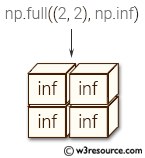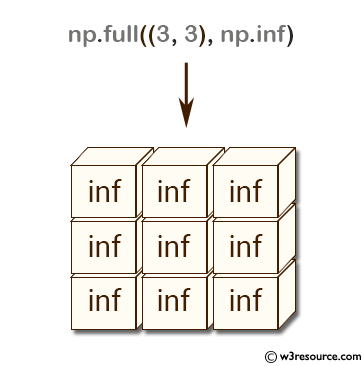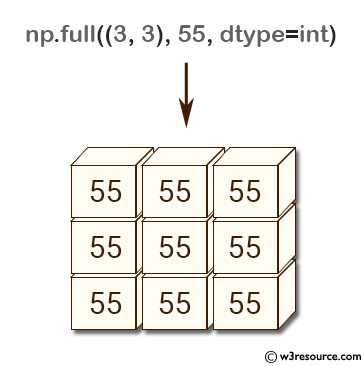# NumPy: numpy.full() function

## numpy.full() function

The numpy.full() function is used to create a new array of the specified shape and type, filled with a specified value.

Syntax:

`numpy.full(shape, fill_value, dtype=None, order='C')`Parameters:

Name Description Required /
Optional
shape Shape of the new array, e.g., (2, 3) or 2. Required
fill_value Fill value. Required
dtype The desired data-type for the array The default, None, means np.array(fill_value).dtype. optional
order Whether to store multidimensional data in C- or Fortran-contiguous (row- or column-wise) order in memory optional

Return value:

[ndarray] Array of fill_value with the given shape, dtype, and order.

Example: Create arrays filled with a constant value using numpy.full()

``````>>> import numpy as np
>>> np.full((3, 3), np.inf)
array([[ inf,  inf,  inf],
[ inf,  inf,  inf],
[ inf,  inf,  inf]])
>>> np.full((3, 3), 10.1)
array([[ 10.1,  10.1,  10.1],
[ 10.1,  10.1,  10.1],
[ 10.1,  10.1,  10.1]])
``````

The above code creates arrays filled with a constant value using the numpy.full() function. In the first example, np.full((3, 3), np.inf) creates a 3x3 numpy array filled with np.inf (infinity). np.inf is a special floating-point value that represents infinity, and is often used in calculations involving limits and asymptotes.
In the second example, np.full((3, 3), 10.1) creates a 3x3 numpy array filled with the value 10.1. Here, the dtype parameter is omitted, so numpy infers the data type of the array from the given value.

Pictorial Presentation:Example: Create an array filled with a single value using np.full()

``````>>> import numpy as np
>>> np.full((3,3), 55, dtype=int)
array([[55, 55, 55],
[55, 55, 55],
[55, 55, 55]])
``````

In the above code, np.full((3,3), 55, dtype=int) creates a 3x3 numpy array filled with the integer value 55. The dtype parameter is explicitly set to int, so the resulting array has integer data type.

Pictorial Presentation:Python - NumPy Code Editor:

Previous: zeros_like()
Next: full_like()

﻿